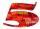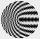PIN code

PIN on Michael credit card is a four-digit number. Michael told this to his friend:
• It is a prime number - that is, a number greater than 1, which is only divisible by number one and by itself.
• The first digit is larger than the second.
• The second digit is greater than the third.
• The third digit is greater than 6.
• The fourth digit is even.
• Three digits are odd.
What is Michael's PIN, if you know that five of the information he gave to a friend is true and one is false?

Result

p =  9871

Solution:Leave us a comment of example and its solution (i.e. if it is still somewhat unclear...):Be the first to comment!To solve this verbal math problem are needed these knowledge from mathematics:

See also our variations calculator. Would you like to compute count of combinations?

Next similar examples:

1. Three digits numberFrom the numbers 1, 2, 3, 4, 5 create three-digit numbers that digits not repeat and number is divisible by 2. How many numbers are there?
2. Toy carsPavel has a collection of toy cars. He wanted to regroup them. But in the division of three, four, six, and eight, he was always one left. Only when he formed groups of seven, he divided everyone. How many toy cars have in the collection?
3. CombinationsHow many different combinations of two-digit number divisible by 4 arises from the digits 3, 5 and 7?
4. NumbersWrite smallest three-digit number, which in division 5 and 7 gives the rest 2.
5. Inverted nineIn the hotel,, Inverted nine" each hotel room number is divisible by 6. How many rooms we can count with three-digit number registered by digits 1,8,7,4,9?
6. DigitsHow many five-digit numbers can be written from numbers 0.3,4, 5, 7 that is divided by 10 and if digits can be repeated.
7. Six-digit primesFind all six-digit prime numbers that contain each one of digits 1,2,4,5,7 and 8 just once. How many are they?In six baskets, the seller has fruit. In individual baskets, there are only apples or just pears with the following number of fruits: 5,6,12,14,23 and 29. "If I sell this basket," the salesman thinks, "then I will have just as many apples as a pear." Which
9. Unknown numberUnknown number is divisible by exactly three different primes. When we compare these primes in ascending order, the following applies: • Difference first and second prime number is half the difference between the third and second prime numbers. • The produ
10. Sum of two primesChristian Goldbach, a mathematician, found out that every even number greater than 2 can be expressed as a sum of two prime numbers. Write or express 2018 as a sum of two prime numbers.
11. Theorem proveWe want to prove the sentence: If the natural number n is divisible by six, then n is divisible by three. From what assumption we started?
12. DivisorsThe sum of all divisors unknown odd number is 2112. Determine sum of all divisors of number which is twice of unknown numbers.
13. Amazing numberAn amazing number is name for such even number, the decomposition product of prime numbers has exactly three not necessarily different factors and the sum of all its divisors is equal to twice that number. Find all amazing numbers.
14. Chebyshev formulaTo estimate the number of primes less than x is used Chebyshev formula: ? Estimate the number of primes less than 30300537.
15. Sheep and cowsThere are only sheep and cows on the farm. Sheep is eight more than cows. The number of cows is half the number of sheep. How many animals live on the farm?
16. TicketsTickets to the zoo cost \$4 for children, \$5 for teenagers and \$6 for adults. In the high season, 1200 people come to the zoo every day. On a certain day, the total revenue at the zoo was \$5300. For every 3 teenagers, 8 children went to the zoo. How many te
17. MedalsIn how many ways can be divided gold, silver and bronze medal among 21 contestant?